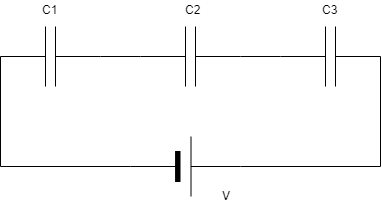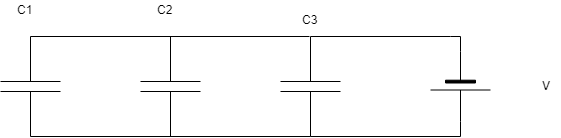# Capacitor in Series and Capacitor in Parallel

In this post, I had explained about the Capacitor in Series and Capacitor in Parallel combination. If you want to know about what Capacitor is and its types, check this post.

Also, read: Resistors in Series & Resistors in Parallel

## Capacitors in Series:

Let Capacitance C1, C2 and C3 be the three capacitors connected in series. Let ‘V’ be the potential difference applied across the series. Let ‘q’ be the charge carried by each capacitor which is connected in series. Let V1, V2, and V3 be the potential difference across the capacitors C1, C2, and C3.

The figure shows the series combination of capacitors.V = V1 + V2 + V3

The potential difference V = q/C

C can be written as Cs since the capacitors are connected in series.

q/Cs = q/C1 + q/C2 + q/C3

q/Cs = q [(1/C1) + (1/C2) + (1/C3)]

1/Cs = (1/C1) + (1/C2) + (1/C3)

(or)

1/Cs = (C2C3 + C1C3 + C1C2)/C1C2C3

(or)

Cs = C1C2C3/ (C2C3 + C1C3 + C1C2)

When ‘n’ number of capacitors are connected in series, the reciprocal of effective capacitance is obtained by the sum of reciprocals of individual capacitors.

Also, Read: Ohms law def | Example Problem also included

## Capacitors in Parallel:

Let three capacitors of capacitances C1, C2 and C3 are connected in parallel. Let ‘V’ be the potential difference of this parallel combination. The Potential difference across each capacitor is the same.The figure shows the parallel combination of the capacitors.

The charges on three capacitors are,

q1 = C1 V

q2 = C2 V

q3 = C3 V

The total charge ‘q’ is

q = q1 + q2 + q3

CV = C1 V + C2 V + C3 V

Since it is a parallel combination we can write C as Cp

Cp V = C1 V + C2 V + C3 V

Cp = C1 + C2 + C3

Also, Read: Calculate | Equivalent Resistance?

Kirchoff’s current law & Kirchoff’s voltage law

When ‘n’ number of capacitors are connected in parallel, the effective capacitance of the parallel combination is equal to the capacitance of sum of the individual Capacitors.

## Have a look at these topics: